The LOGISTIC Procedure
 EXACT Statement
EXACT <’label’> <INTERCEPT> <effects> </ options> ;

The EXACT statement performs exact tests of the parameters for the specified effects and optionally estimates the parameters and outputs the exact conditional distributions. You can specify the keyword INTERCEPT and any effects in the MODEL statement. Inference on the parameters of the specified effects is performed by conditioning on the sufficient statistics of all the other model parameters (possibly including the intercept).

You can specify several EXACT statements, but they must follow the MODEL statement. Each statement can optionally include an identifying label. If several EXACT statements are specified, any statement without a label is assigned a label of the form "Exact," whereindicates theth EXACT statement. The label is included in the headers of the displayed exact analysis tables.

If a STRATA statement is also specified, then a stratified exact logistic regression is performed. The model contains a different intercept for each stratum, and these intercepts are conditioned out of the model along with any other nuisance parameters (parameters for effects specified in the MODEL statement that are not in the EXACT statement).

If the LINK=GLOGIT option is specified in the MODEL statement, then the METHOD=DIRECT option is invoked in the EXACTOPTIONS statement by default and a generalized logit model is fit. Since each effect specified in the MODEL statement addsparameters to the model (where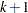is the number of response levels), exact analysis of the generalized logit model by using this method is limited to rather small problems.

The CONTRAST, ESTIMATE, LSMEANS, LSMESTIMATE, ODDSRATIO, OUTPUT, ROC, ROCCONTRAST, SCORE, SLICE, STORE, TEST, and UNITS statements are not available with an exact analysis. Exact analyses are not performed when you specify a WEIGHT statement, a link other than LINK=LOGIT or LINK=GLOGIT, an offset variable, the NOFIT option, or a model selection method. Exact estimation is not available for ordinal response models.

For classification variables, use of the reference parameterization is recommended.

The following options can be specified in each EXACT statement after a slash (/):

ALPHA=number

specifies the level of significancefor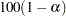% confidence limits for the parameters or odds ratios. The value of number must be between 0 and 1. By default, number is equal to the value of the ALPHA= option in the PROC LOGISTIC statement, or 0.05 if that option is not specified.

CLTYPE=EXACT | MIDP

requests either the exact or mid-confidence intervals for the parameter estimates. By default, the exact intervals are produced. The confidence coefficient can be specified with the ALPHA= option. The mid-interval can be modified with the MIDPFACTOR= option. See the section Exact Conditional Logistic Regression for details.

ESTIMATE <=keyword>

estimates the individual parameters (conditioned on all other parameters) for the effects specified in the EXACT statement. For each parameter, a point estimate, a standard error, a confidence interval, and a p-value for a two-sided test that the parameter is zero are displayed. Note that the two-sided p-value is twice the one-sided p-value. You can optionally specify one of the following keywords:

PARM

specifies that the parameters be estimated. This is the default.

ODDS

specifies that the odds ratios be estimated. If you have classification variables, then you must also specify the PARAM=REF option in the CLASS statement.

BOTH

specifies that both the parameters and odds ratios be estimated.

JOINT

performs the joint test that all of the parameters are simultaneously equal to zero, performs individual hypothesis tests for the parameter of each continuous variable, and performs joint tests for the parameters of each classification variable. The joint test is indicated in the "Conditional Exact Tests" table by the label "Joint."

JOINTONLY

performs only the joint test of the parameters. The test is indicated in the "Conditional Exact Tests" table by the label "Joint." When this option is specified, individual tests for the parameters of each continuous variable and joint tests for the parameters of the classification variables are not performed.

MIDPFACTOR=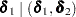sets the tie factors used to produce the mid-hypothesis statistics and the mid-confidence intervals.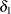modifies both the hypothesis tests and confidence intervals, while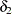affects only the hypothesis tests. By default,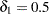and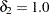. See the section Exact Conditional Logistic Regression for details.

ONESIDED

requests one-sided confidence intervals and p-values for the individual parameter estimates and odds ratios. The one-sided p-value is the smaller of the left- and right-tail probabilities for the observed sufficient statistic of the parameter under the null hypothesis that the parameter is zero. The two-sided p-values (default) are twice the one-sided p-values. See the section Exact Conditional Logistic Regression for more details.

OUTDIST=SAS-data-set

names the SAS data set that contains the exact conditional distributions. This data set contains all of the exact conditional distributions that are required to process the corresponding EXACT statement. This data set contains the possible sufficient statistics for the parameters of the effects specified in the EXACT statement, the counts, and, when hypothesis tests are performed on the parameters, the probability of occurrence and the score value for each sufficient statistic. When you request an OUTDIST= data set, the observed sufficient statistics are displayed in the "Sufficient Statistics" table. See the section OUTDIST= Output Data Set for more information.

EXACT Statement Examples

In the following example, two exact tests are computed: one for x1 and the other for x2. The test for x1 is based on the exact conditional distribution of the sufficient statistic for the x1 parameter given the observed values of the sufficient statistics for the intercept, x2, and x3 parameters; likewise, the test for x2 is conditional on the observed sufficient statistics for the intercept, x1, and x3.

proc logistic;
model y= x1 x2 x3;
exact x1 x2;
run;

PROC LOGISTIC determines, from all the specified EXACT statements, the distinct conditional distributions that need to be evaluated. For example, there is only one exact conditional distribution for the following two EXACT statements:

exact 'One' x1 / estimate=parm;
exact 'Two' x1 / estimate=parm onesided;

For each EXACT statement, individual tests for the parameters of the specified effects are computed unless the JOINTONLY option is specified. Consider the following EXACT statements:

exact 'E12' x1 x2 / estimate;
exact 'E1'  x1    / estimate;
exact 'E2'  x2    / estimate;
exact 'J12' x1 x2 / joint;

In the E12 statement, the parameters for x1 and x2 are estimated and tested separately. Specifying the E12 statement is equivalent to specifying both the E1 and E2 statements. In the J12 statement, the joint test for the parameters of x1 and x2 is computed in addition to the individual tests for x1 and x2.Previous Page | Next Page | Top of Page